## Example Questions

### Example Question #61 : Trigonometry

Which of the following statements is (are) true:

I. The domain of the tangent function is all real numbers

II. The range of the sine function is all real numbers

III. The periods of the tangent, sine, and cosine functions are the same.

II only

II and III only

none of the above

I and II only

I only

II only

Explanation:

The domain of the tangent function does not include any values of x that are odd multiples of π/2 .

The range of the sine function is from [-1, 1].

The period of the tangent function is π, whereas the period for both sine and cosine is 2π.

### Example Question #62 : Trigonometry

Which of the following represents a sine wave with a range of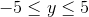?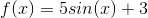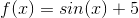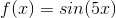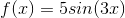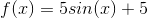Explanation:

The range of a sine wave is altered by the coefficient placed in front of the base equation. So, if you have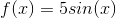, this means that the highest point on the wave will be atand the lowest at.  However, if you then begin to shift the equation vertically by adding values, as in,, then you need to account for said shift.  This would make the minimum value to beand the maximum value to be. For our question, then, it is fine to use. Thefor the function parameter only alters the period of the equation, making its waves "thinner."

### Example Question #63 : Trigonometry

Which of the following sine waves has a range ofto?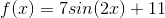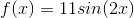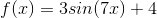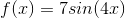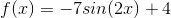Explanation:

The range of a sine wave is altered by the coefficient placed in front of the base equation. So, if you have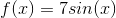, this means that the highest point on the wave will be atand the lowest at; however, if you then begin to shift the equation vertically by adding values, as in,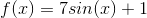, then you need to account for said shift. This would make the minimum value to beand the maximum value to be.

For our question, the range of values covers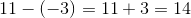. This range is accomplished by having eitheroras your coefficient. (merely flips the equation over the-axis. The range "spread" remains the same.) We need to make the upper value to beinstead of. To do this, you will need to addto. This requires an upward shift of.  An example of performing a shift like this is: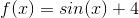Among the possible answers, the one that works is:Theparameter does not matter, as it only alters the frequency of the function.

### Example Question #64 : Trigonometry

What is the range of the trigonometric function given by the equation: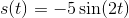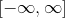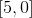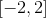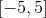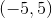Explanation:

The range of the sine and cosine functions are the closed interval from the negative amplitude and the positive amplitude. The amplitude is given by the coefficient,in the following general equation: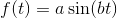. Thus we see the range is:### Example Question #65 : Trigonometry

What is the range of the following trigonometric equation: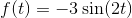?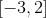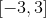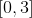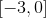The range of a sine or cosine function spans from the negative amplitude to the positive amplitude. The amplitude isin the general formula:Thus we see amplitude of our function isand so the range is: